# Parallel algorithm in linear algebra

18. Nov 2015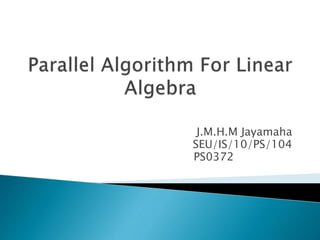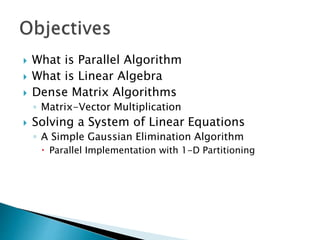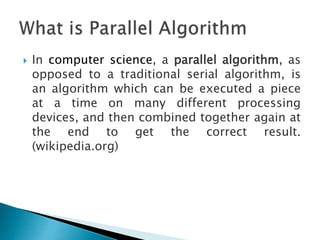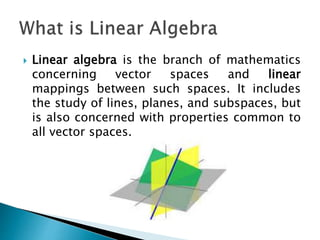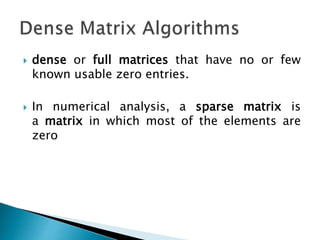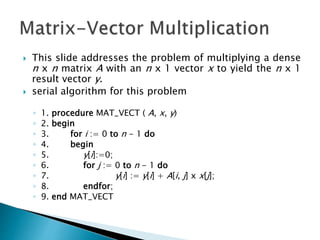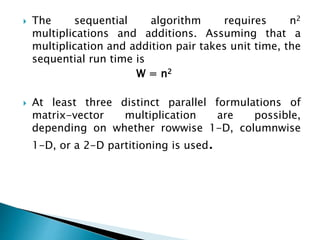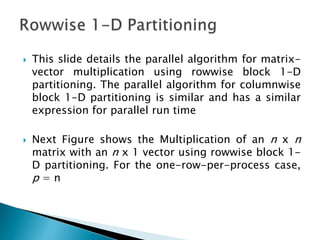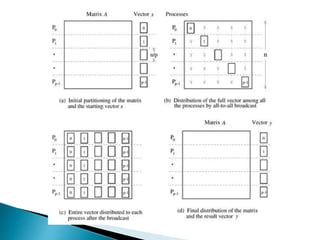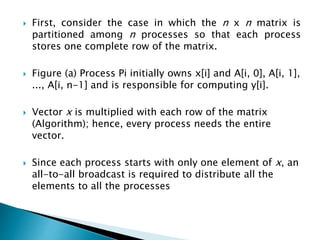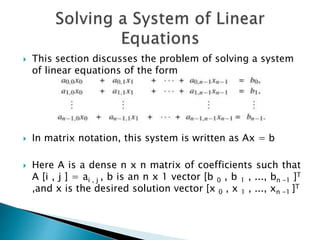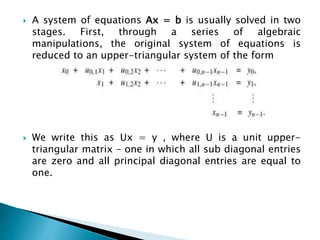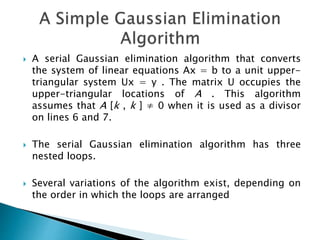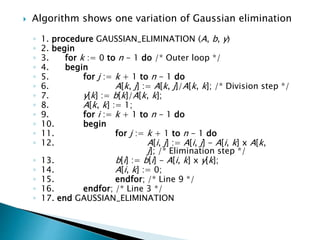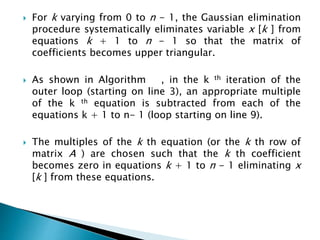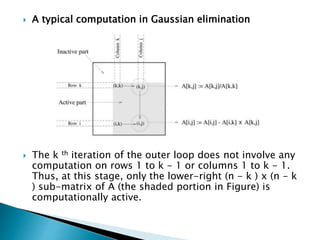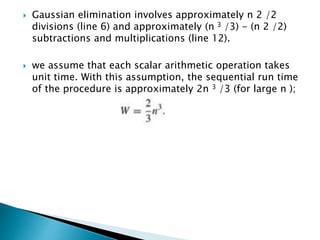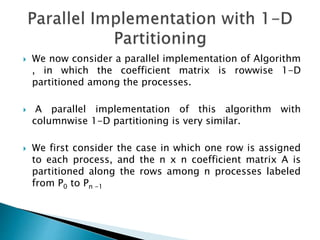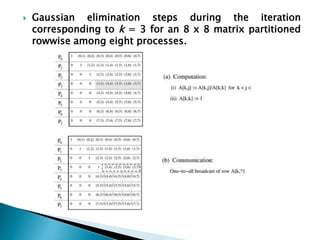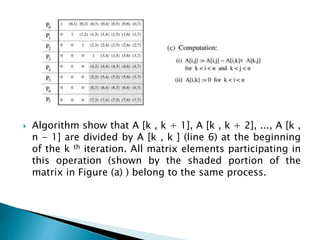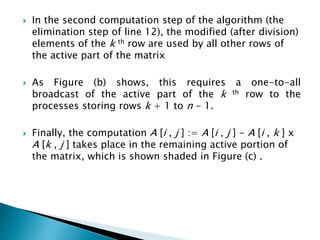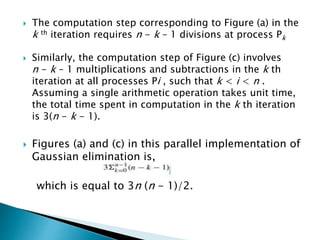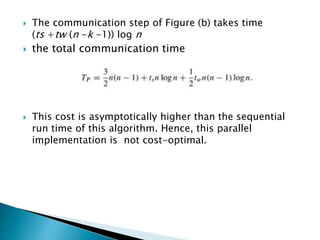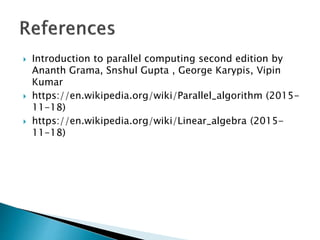1 von 26

### Parallel algorithm in linear algebra

• 1. J.M.H.M Jayamaha SEU/IS/10/PS/104 PS0372
• 2.  What is Parallel Algorithm  What is Linear Algebra  Dense Matrix Algorithms ◦ Matrix-Vector Multiplication  Solving a System of Linear Equations ◦ A Simple Gaussian Elimination Algorithm  Parallel Implementation with 1-D Partitioning
• 3.  In computer science, a parallel algorithm, as opposed to a traditional serial algorithm, is an algorithm which can be executed a piece at a time on many different processing devices, and then combined together again at the end to get the correct result. (wikipedia.org)
• 4.  Linear algebra is the branch of mathematics concerning vector spaces and linear mappings between such spaces. It includes the study of lines, planes, and subspaces, but is also concerned with properties common to all vector spaces.
• 5.  dense or full matrices that have no or few known usable zero entries.  In numerical analysis, a sparse matrix is a matrix in which most of the elements are zero
• 6.  This slide addresses the problem of multiplying a dense n x n matrix A with an n x 1 vector x to yield the n x 1 result vector y.  serial algorithm for this problem ◦ 1. procedure MAT_VECT ( A, x, y) ◦ 2. begin ◦ 3. for i := 0 to n - 1 do ◦ 4. begin ◦ 5. y[i]:=0; ◦ 6. for j := 0 to n - 1 do ◦ 7. y[i] := y[i] + A[i, j] x x[j]; ◦ 8. endfor; ◦ 9. end MAT_VECT
• 7.  The sequential algorithm requires n2 multiplications and additions. Assuming that a multiplication and addition pair takes unit time, the sequential run time is W = n2  At least three distinct parallel formulations of matrix-vector multiplication are possible, depending on whether rowwise 1-D, columnwise 1-D, or a 2-D partitioning is used.
• 8.  This slide details the parallel algorithm for matrix- vector multiplication using rowwise block 1-D partitioning. The parallel algorithm for columnwise block 1-D partitioning is similar and has a similar expression for parallel run time  Next Figure shows the Multiplication of an n x n matrix with an n x 1 vector using rowwise block 1- D partitioning. For the one-row-per-process case, p = n
• 10.  First, consider the case in which the n x n matrix is partitioned among n processes so that each process stores one complete row of the matrix.  Figure (a) Process Pi initially owns x[i] and A[i, 0], A[i, 1], ..., A[i, n-1] and is responsible for computing y[i].  Vector x is multiplied with each row of the matrix (Algorithm); hence, every process needs the entire vector.  Since each process starts with only one element of x, an all-to-all broadcast is required to distribute all the elements to all the processes
• 11.  This section discusses the problem of solving a system of linear equations of the form  In matrix notation, this system is written as Ax = b  Here A is a dense n x n matrix of coefficients such that A [i , j ] = ai , j , b is an n x 1 vector [b 0 , b 1 , ..., bn -1 ]T ,and x is the desired solution vector [x 0 , x 1 , ..., xn -1 ]T
• 12.  A system of equations Ax = b is usually solved in two stages. First, through a series of algebraic manipulations, the original system of equations is reduced to an upper-triangular system of the form  We write this as Ux = y , where U is a unit upper- triangular matrix – one in which all sub diagonal entries are zero and all principal diagonal entries are equal to one.
• 13.  A serial Gaussian elimination algorithm that converts the system of linear equations Ax = b to a unit upper- triangular system Ux = y . The matrix U occupies the upper-triangular locations of A . This algorithm assumes that A [k , k ] ≠ 0 when it is used as a divisor on lines 6 and 7.  The serial Gaussian elimination algorithm has three nested loops.  Several variations of the algorithm exist, depending on the order in which the loops are arranged
• 14.  Algorithm shows one variation of Gaussian elimination ◦ 1. procedure GAUSSIAN_ELIMINATION (A, b, y) ◦ 2. begin ◦ 3. for k := 0 to n - 1 do /* Outer loop */ ◦ 4. begin ◦ 5. for j := k + 1 to n - 1 do ◦ 6. A[k, j] := A[k, j]/A[k, k]; /* Division step */ ◦ 7. y[k] := b[k]/A[k, k]; ◦ 8. A[k, k] := 1; ◦ 9. for i := k + 1 to n - 1 do ◦ 10. begin ◦ 11. for j := k + 1 to n - 1 do ◦ 12. A[i, j] := A[i, j] - A[i, k] x A[k, j]; /* Elimination step */ ◦ 13. b[i] := b[i] - A[i, k] x y[k]; ◦ 14. A[i, k] := 0; ◦ 15. endfor; /* Line 9 */ ◦ 16. endfor; /* Line 3 */ ◦ 17. end GAUSSIAN_ELIMINATION
• 15.  For k varying from 0 to n - 1, the Gaussian elimination procedure systematically eliminates variable x [k ] from equations k + 1 to n - 1 so that the matrix of coefficients becomes upper triangular.  As shown in Algorithm , in the k th iteration of the outer loop (starting on line 3), an appropriate multiple of the k th equation is subtracted from each of the equations k + 1 to n- 1 (loop starting on line 9).  The multiples of the k th equation (or the k th row of matrix A ) are chosen such that the k th coefficient becomes zero in equations k + 1 to n - 1 eliminating x [k ] from these equations.
• 16.  A typical computation in Gaussian elimination  The k th iteration of the outer loop does not involve any computation on rows 1 to k - 1 or columns 1 to k - 1. Thus, at this stage, only the lower-right (n - k ) x (n - k ) sub-matrix of A (the shaded portion in Figure) is computationally active.
• 17.  Gaussian elimination involves approximately n 2 /2 divisions (line 6) and approximately (n 3 /3) - (n 2 /2) subtractions and multiplications (line 12).  we assume that each scalar arithmetic operation takes unit time. With this assumption, the sequential run time of the procedure is approximately 2n 3 /3 (for large n );
• 18.  We now consider a parallel implementation of Algorithm , in which the coefficient matrix is rowwise 1-D partitioned among the processes.  A parallel implementation of this algorithm with columnwise 1-D partitioning is very similar.  We first consider the case in which one row is assigned to each process, and the n x n coefficient matrix A is partitioned along the rows among n processes labeled from P0 to Pn -1
• 19.  Gaussian elimination steps during the iteration corresponding to k = 3 for an 8 x 8 matrix partitioned rowwise among eight processes.
• 20.  Algorithm show that A [k , k + 1], A [k , k + 2], ..., A [k , n - 1] are divided by A [k , k ] (line 6) at the beginning of the k th iteration. All matrix elements participating in this operation (shown by the shaded portion of the matrix in Figure (a) ) belong to the same process.
• 21.  In the second computation step of the algorithm (the elimination step of line 12), the modified (after division) elements of the k th row are used by all other rows of the active part of the matrix  As Figure (b) shows, this requires a one-to-all broadcast of the active part of the k th row to the processes storing rows k + 1 to n - 1.  Finally, the computation A [i , j ] := A [i , j ] - A [i , k ] x A [k , j ] takes place in the remaining active portion of the matrix, which is shown shaded in Figure (c) .
• 22.  The computation step corresponding to Figure (a) in the k th iteration requires n - k – 1 divisions at process Pk  Similarly, the computation step of Figure (c) involves n - k – 1 multiplications and subtractions in the k th iteration at all processes Pi , such that k < i < n . Assuming a single arithmetic operation takes unit time, the total time spent in computation in the k th iteration is 3(n - k - 1).  Figures (a) and (c) in this parallel implementation of Gaussian elimination is, which is equal to 3n (n - 1)/2.
• 23.  The communication step of Figure (b) takes time (ts +tw (n -k -1)) log n  the total communication time  This cost is asymptotically higher than the sequential run time of this algorithm. Hence, this parallel implementation is not cost-optimal.
• 24.  Introduction to parallel computing second edition by Ananth Grama, Snshul Gupta , George Karypis, Vipin Kumar  https://en.wikipedia.org/wiki/Parallel_algorithm (2015- 11-18)  https://en.wikipedia.org/wiki/Linear_algebra (2015- 11-18)